Successfully reported this slideshow.
We use your LinkedIn profile and activity data to personalize ads and to show you more relevant ads. You can change your ad preferences anytime.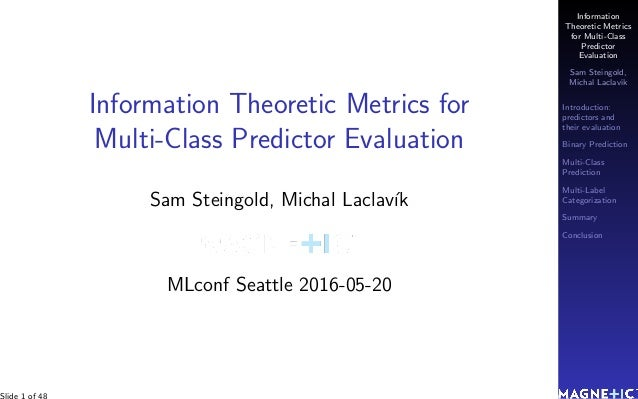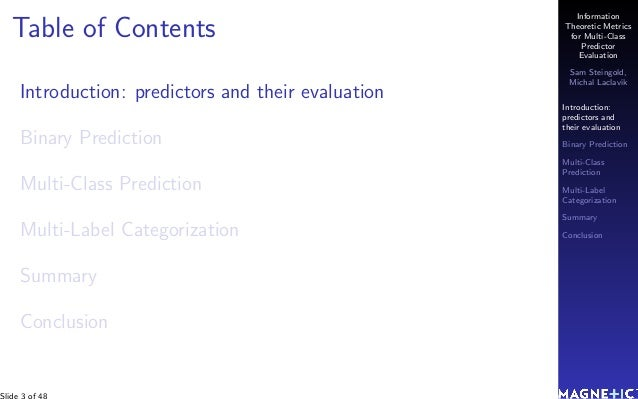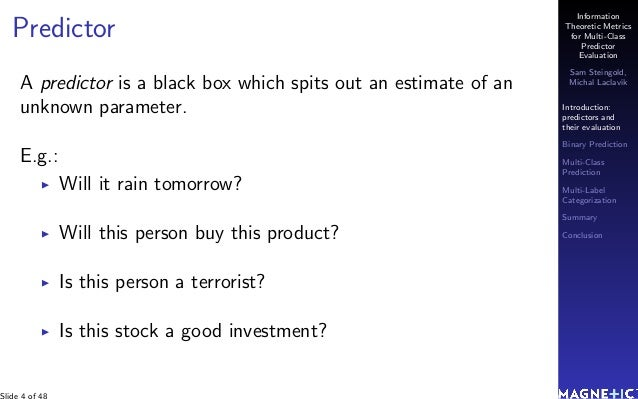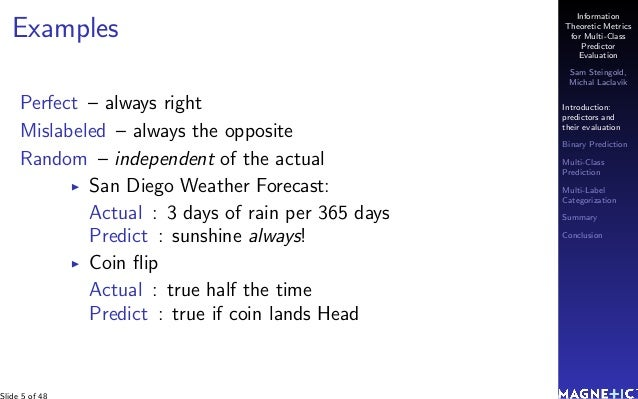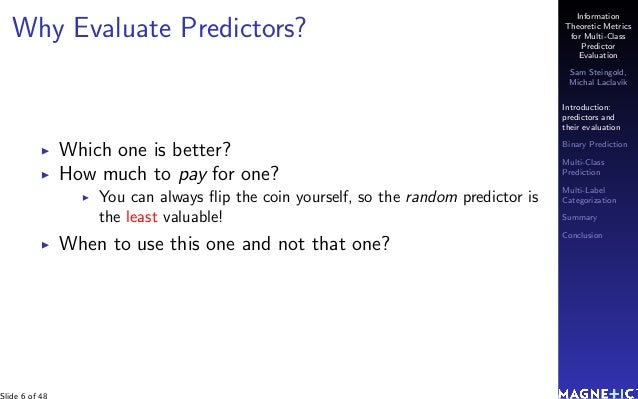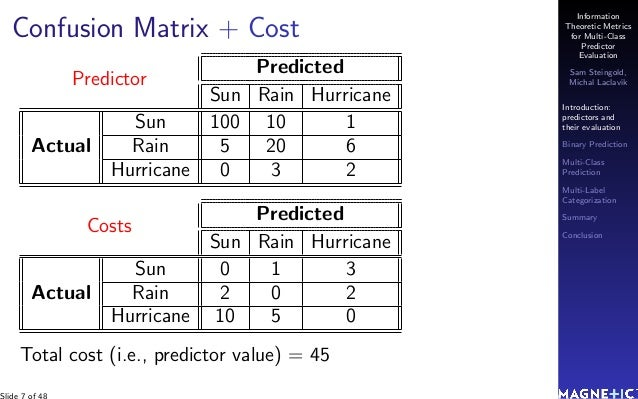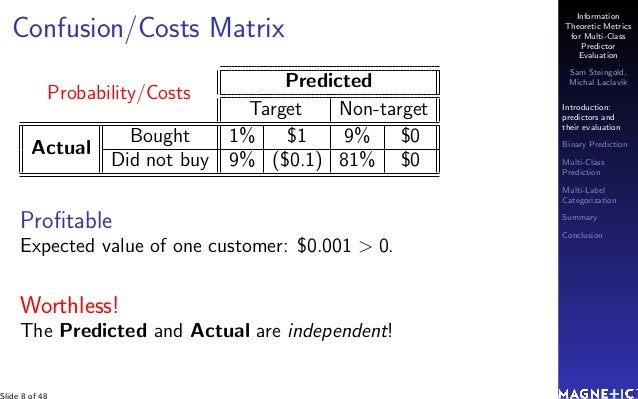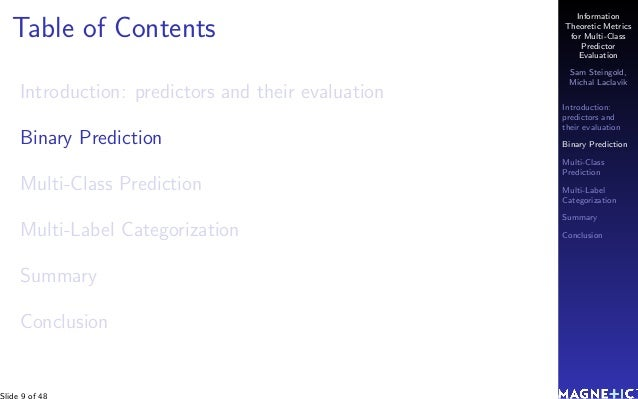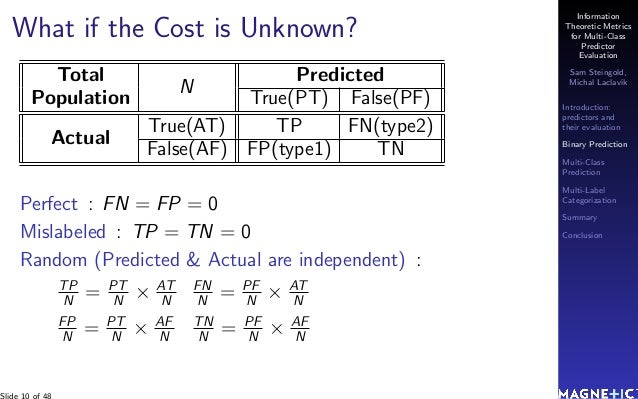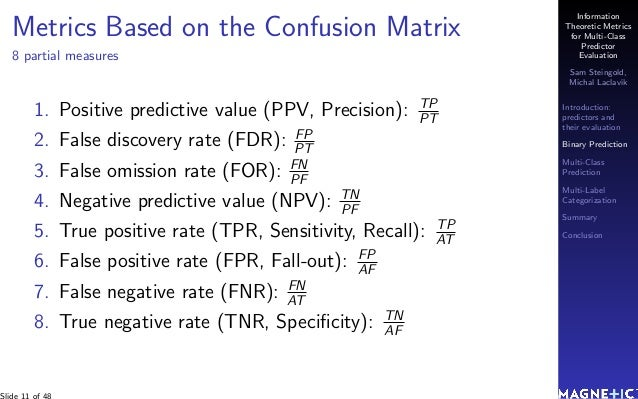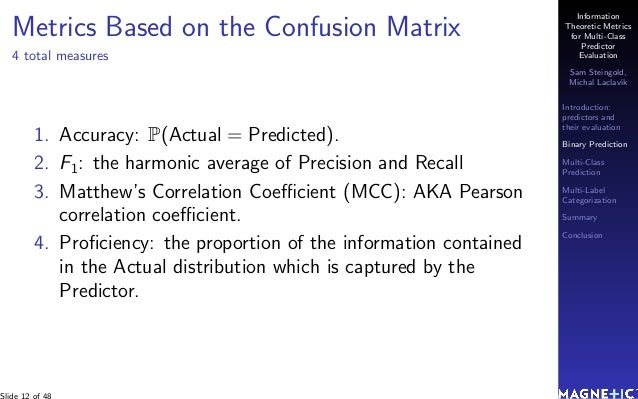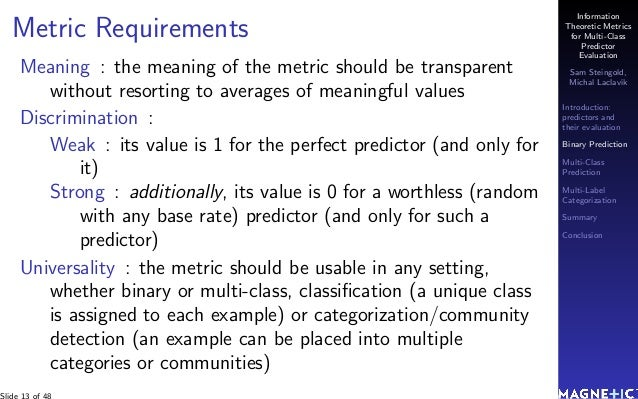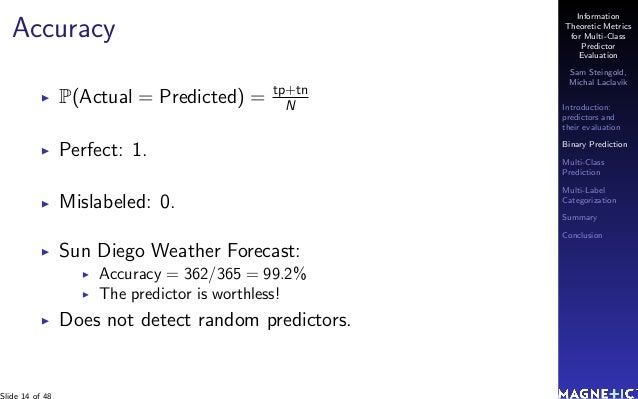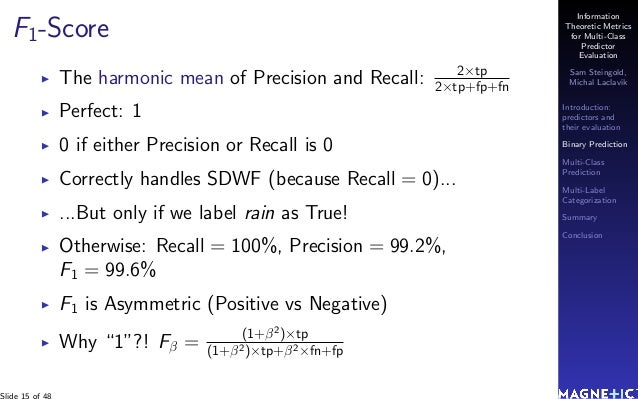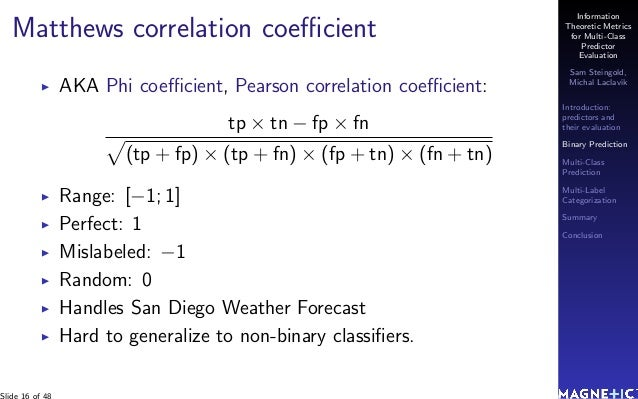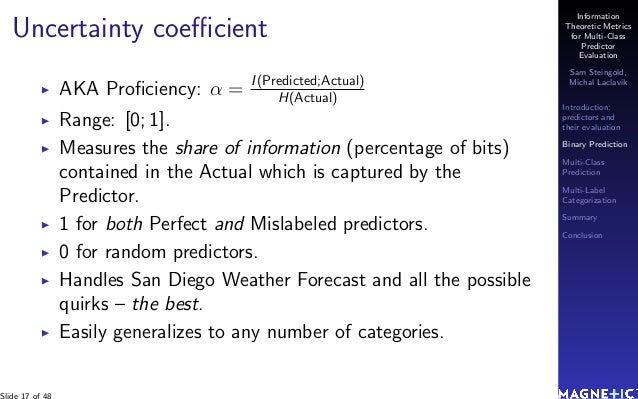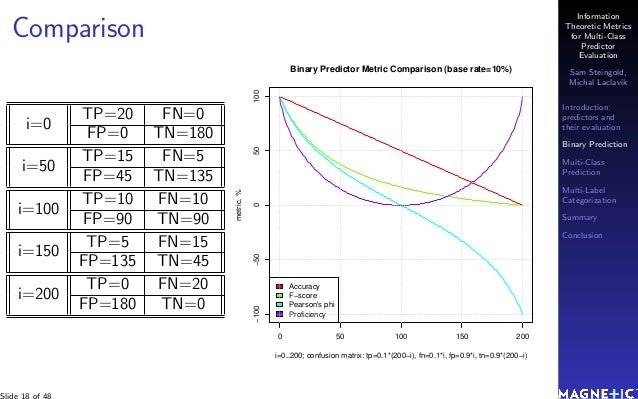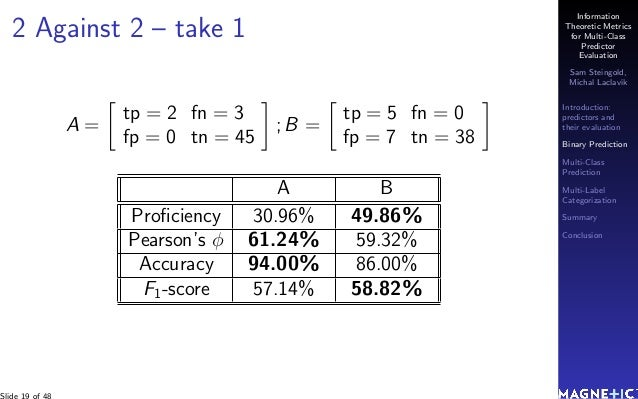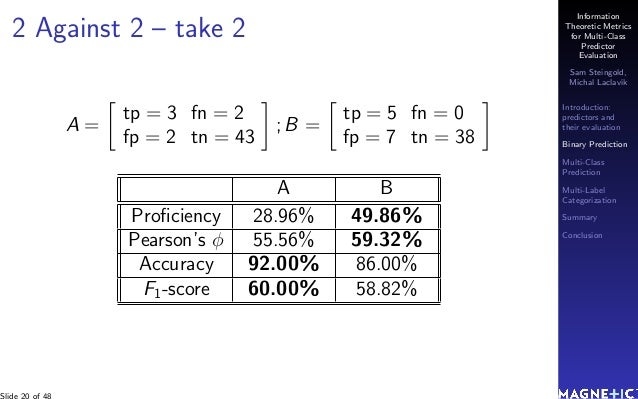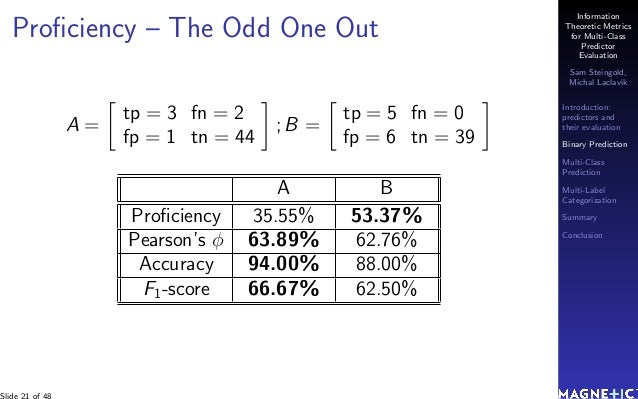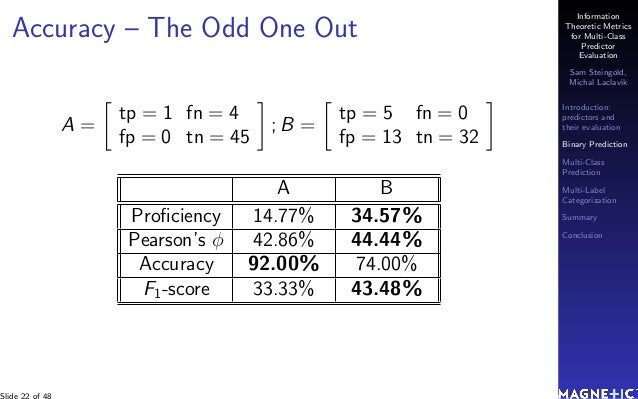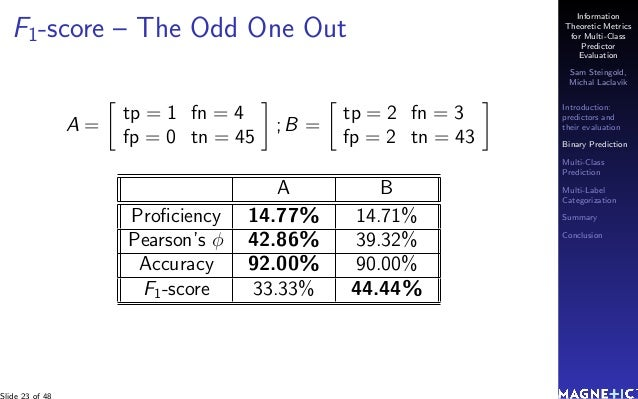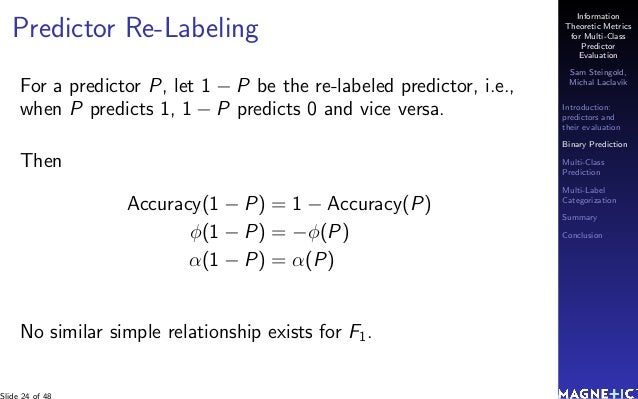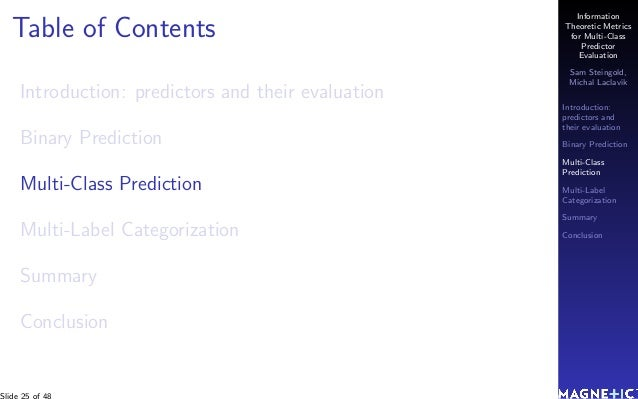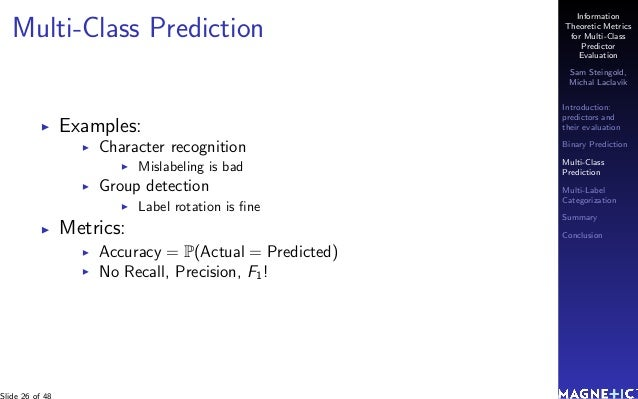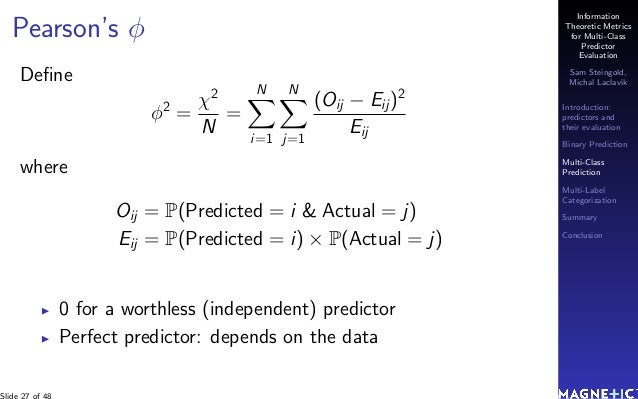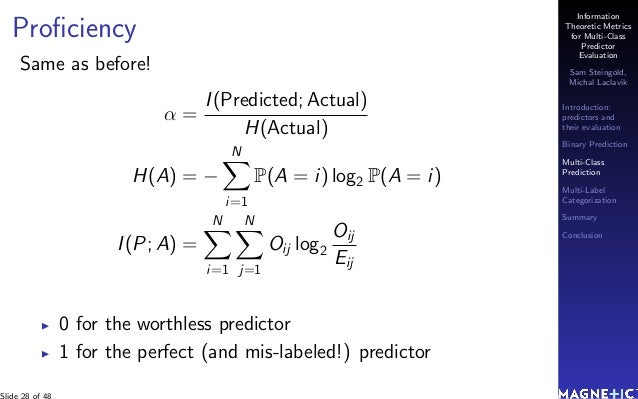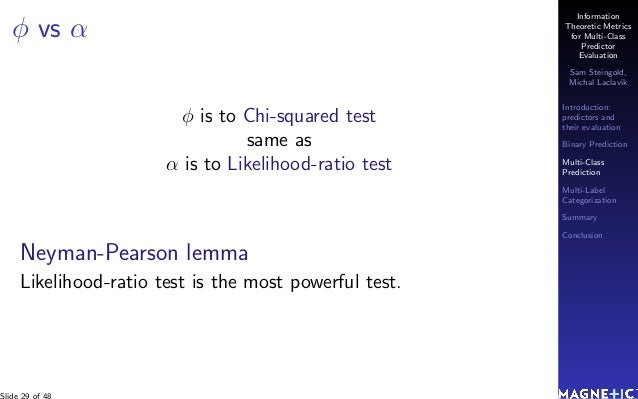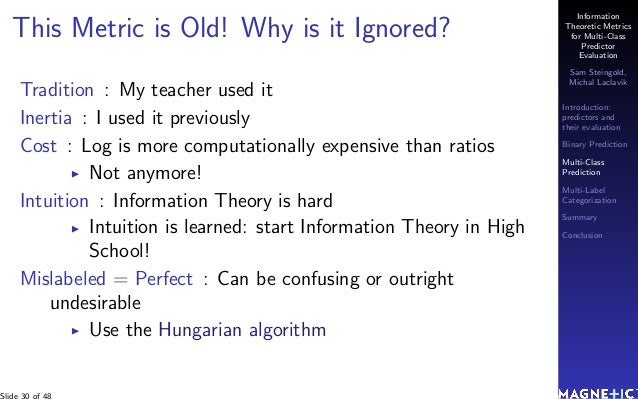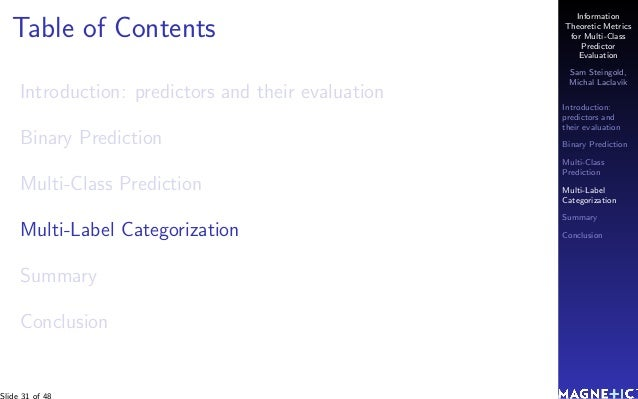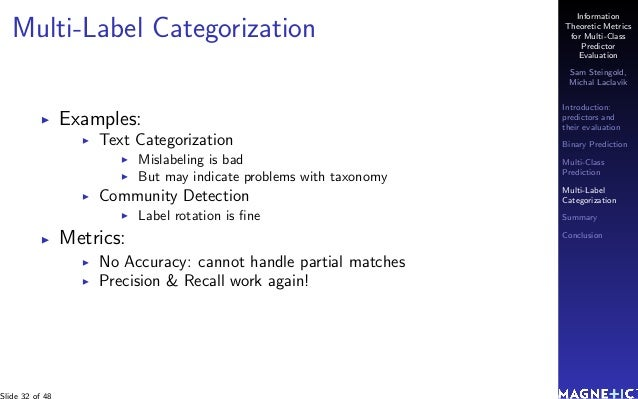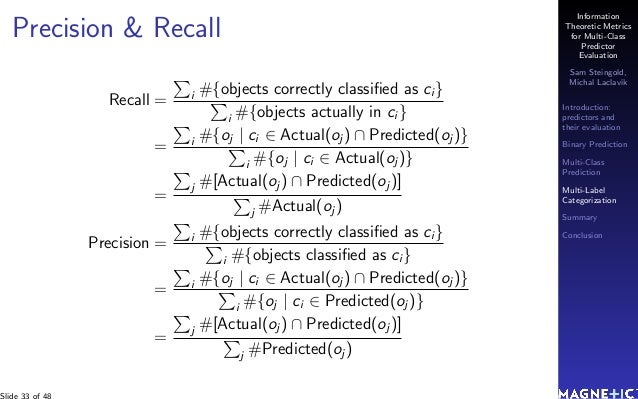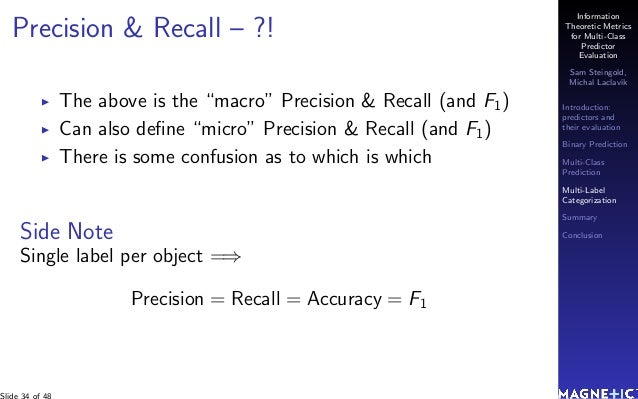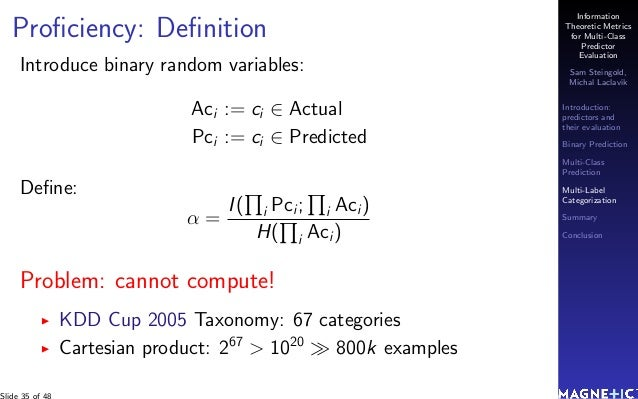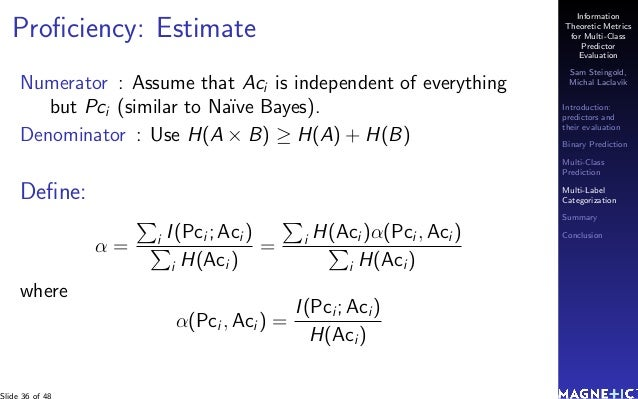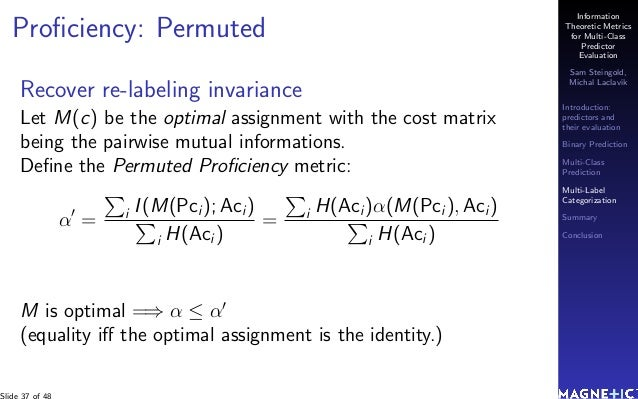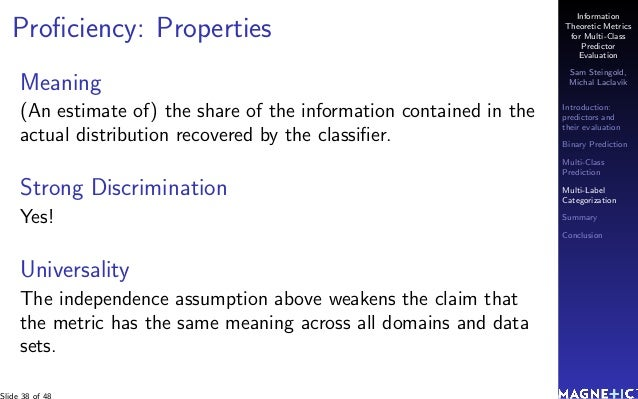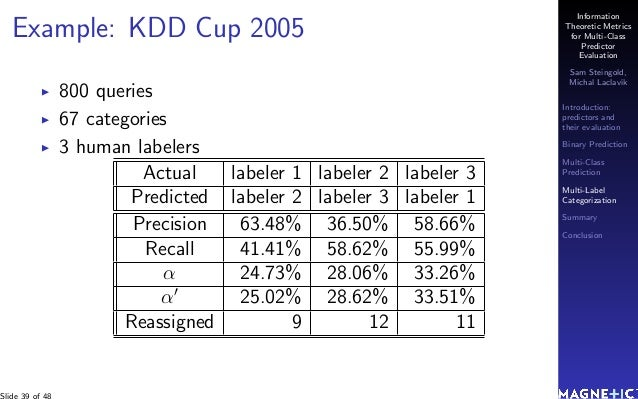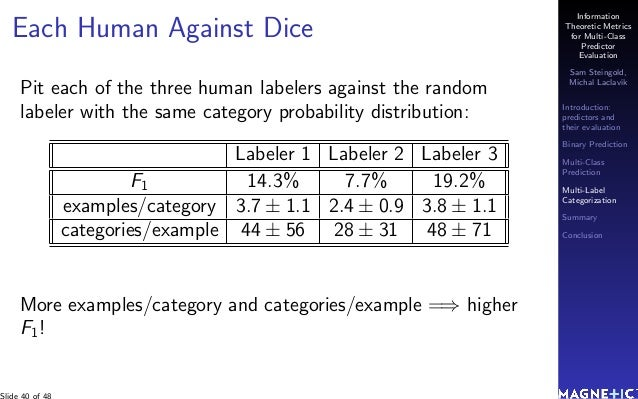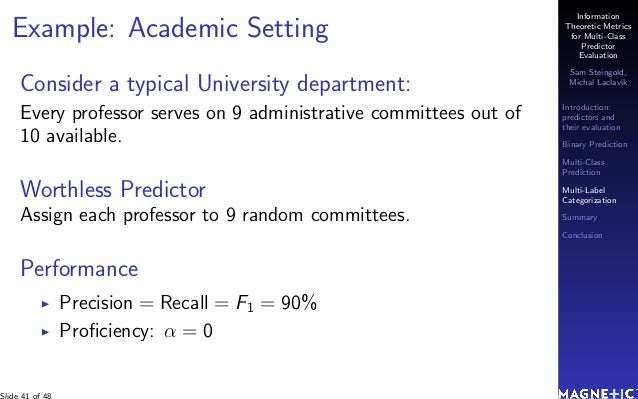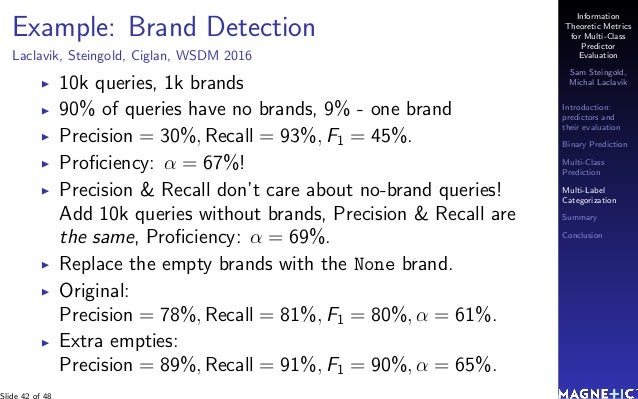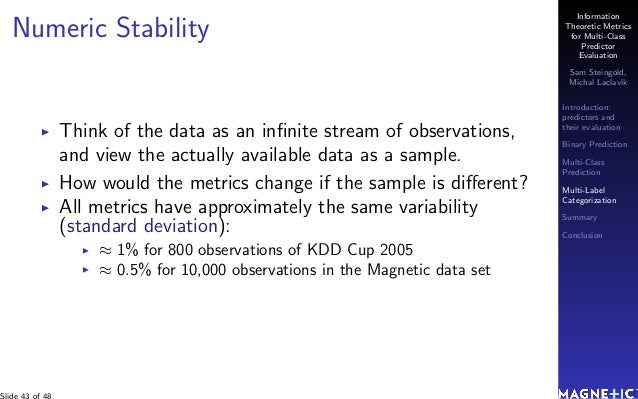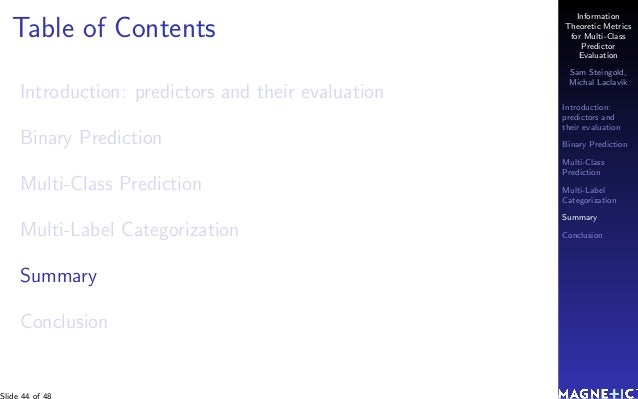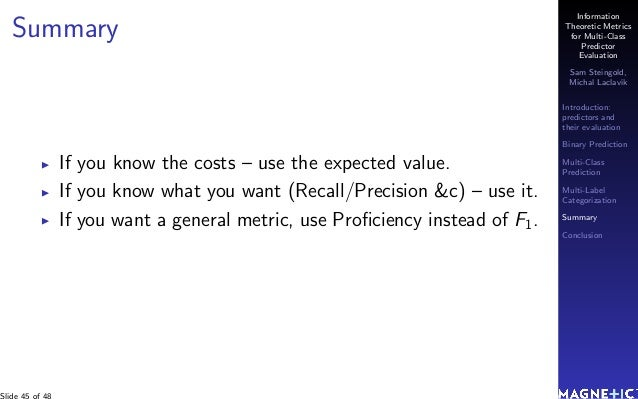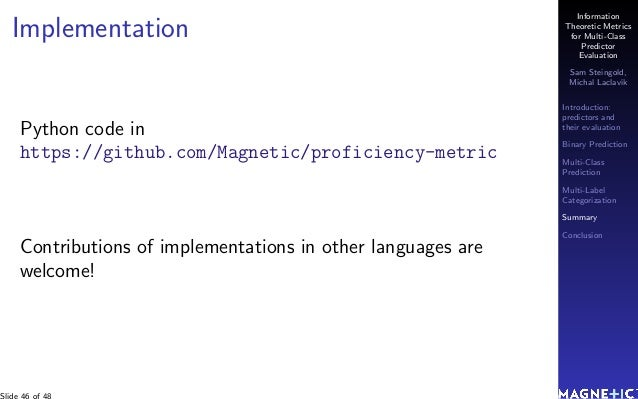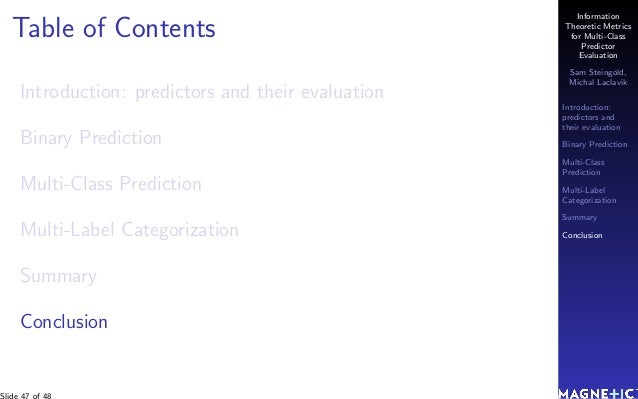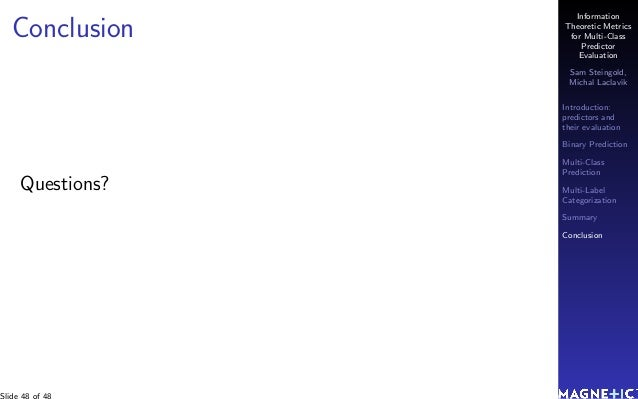Upcoming SlideShare
Loading in …5
×

# Sam Steingold, Lead Data Scientist, Magnetic Media Online at MLconf SEA - 5/20/16

An Information Theoretic Metric for Multi-Class Categorization: The most common metrics used to evaluate a classifier are accuracy, recall and precision, and \$F_1\$-score. These metrics are widely used in machine learning, information retrieval, and text analysis (e.g., text categorization). Each of these metrics is imperfect in some way (captures only one aspect of predictor performance and can be fooled by a weird data set). None of them can be used to compare predictors across different datasets. In this paper we present an information-theoretic performance metric which does not suffer from the aforementioned flaws and can be used in both classification (binary and multi-class) and categorization (each example can be placed in several categories) settings. The code to compute the metric is available under the Apache open-source license.

• Full Name
Comment goes here.

Are you sure you want to Yes No
Your message goes here• Be the first to comment

### Sam Steingold, Lead Data Scientist, Magnetic Media Online at MLconf SEA - 5/20/16

1. 1. Information Theoretic Metrics for Multi-Class Predictor Evaluation Sam Steingold, Michal Laclav´ık Introduction: predictors and their evaluation Binary Prediction Multi-Class Prediction Multi-Label Categorization Summary Conclusion Information Theoretic Metrics for Multi-Class Predictor Evaluation Sam Steingold, Michal Laclav´ık MLconf Seattle 2016-05-20 Slide 1 of 48
2. 2. Information Theoretic Metrics for Multi-Class Predictor Evaluation Sam Steingold, Michal Laclav´ık Introduction: predictors and their evaluation Binary Prediction Multi-Class Prediction Multi-Label Categorization Summary Conclusion Who We Are: A Fast Growing Startup One of the fastest growing tech startups – 50% YoY growth. Marketing Tech Platform: Email + Site + Ads + Mobile 700+ customers in Retail, Auto, Travel, CPG, Finance Four Engineering locations: NY, SF, London, Ann Arbor 275+ employees. We are hiring aggressively! Slide 2 of 48
3. 3. Information Theoretic Metrics for Multi-Class Predictor Evaluation Sam Steingold, Michal Laclav´ık Introduction: predictors and their evaluation Binary Prediction Multi-Class Prediction Multi-Label Categorization Summary Conclusion Table of Contents Introduction: predictors and their evaluation Binary Prediction Multi-Class Prediction Multi-Label Categorization Summary Conclusion Slide 3 of 48
4. 4. Information Theoretic Metrics for Multi-Class Predictor Evaluation Sam Steingold, Michal Laclav´ık Introduction: predictors and their evaluation Binary Prediction Multi-Class Prediction Multi-Label Categorization Summary Conclusion Predictor A predictor is a black box which spits out an estimate of an unknown parameter. E.g.: Will it rain tomorrow? Will this person buy this product? Is this person a terrorist? Is this stock a good investment?
5. 5. Information Theoretic Metrics for Multi-Class Predictor Evaluation Sam Steingold, Michal Laclav´ık Introduction: predictors and their evaluation Binary Prediction Multi-Class Prediction Multi-Label Categorization Summary Conclusion Examples Perfect – always right Mislabeled – always the opposite Random – independent of the actual San Diego Weather Forecast: Actual : 3 days of rain per 365 days Predict : sunshine always! Coin ﬂip Actual : true half the time Predict : true if coin lands Head Slide 5 of 48
6. 6. Information Theoretic Metrics for Multi-Class Predictor Evaluation Sam Steingold, Michal Laclav´ık Introduction: predictors and their evaluation Binary Prediction Multi-Class Prediction Multi-Label Categorization Summary Conclusion Why Evaluate Predictors? Which one is better? How much to pay for one? You can always ﬂip the coin yourself, so the random predictor is the least valuable! When to use this one and not that one? Slide 6 of 48
7. 7. Information Theoretic Metrics for Multi-Class Predictor Evaluation Sam Steingold, Michal Laclav´ık Introduction: predictors and their evaluation Binary Prediction Multi-Class Prediction Multi-Label Categorization Summary Conclusion Confusion Matrix + Cost Predictor Predicted Sun Rain Hurricane Actual Sun 100 10 1 Rain 5 20 6 Hurricane 0 3 2 Costs Predicted Sun Rain Hurricane Actual Sun 0 1 3 Rain 2 0 2 Hurricane 10 5 0 Total cost (i.e., predictor value) = 45 Slide 7 of 48
8. 8. Information Theoretic Metrics for Multi-Class Predictor Evaluation Sam Steingold, Michal Laclav´ık Introduction: predictors and their evaluation Binary Prediction Multi-Class Prediction Multi-Label Categorization Summary Conclusion Confusion/Costs Matrix Probability/Costs Predicted Target Non-target Actual Bought 1% \$1 9% \$0 Did not buy 9% (\$0.1) 81% \$0 Proﬁtable Expected value of one customer: \$0.001 > 0. Worthless! The Predicted and Actual are independent!
9. 9. Information Theoretic Metrics for Multi-Class Predictor Evaluation Sam Steingold, Michal Laclav´ık Introduction: predictors and their evaluation Binary Prediction Multi-Class Prediction Multi-Label Categorization Summary Conclusion Table of Contents Introduction: predictors and their evaluation Binary Prediction Multi-Class Prediction Multi-Label Categorization Summary Conclusion Slide 9 of 48
10. 10. Information Theoretic Metrics for Multi-Class Predictor Evaluation Sam Steingold, Michal Laclav´ık Introduction: predictors and their evaluation Binary Prediction Multi-Class Prediction Multi-Label Categorization Summary Conclusion What if the Cost is Unknown? Total N Predicted Population True(PT) False(PF) Actual True(AT) TP FN(type2) False(AF) FP(type1) TN Perfect : FN = FP = 0 Mislabeled : TP = TN = 0 Random (Predicted & Actual are independent) : TP N = PT N × AT N FN N = PF N × AT N FP N = PT N × AF N TN N = PF N × AF N
11. 11. Information Theoretic Metrics for Multi-Class Predictor Evaluation Sam Steingold, Michal Laclav´ık Introduction: predictors and their evaluation Binary Prediction Multi-Class Prediction Multi-Label Categorization Summary Conclusion Metrics Based on the Confusion Matrix 8 partial measures 1. Positive predictive value (PPV, Precision): TP PT 2. False discovery rate (FDR): FP PT 3. False omission rate (FOR): FN PF 4. Negative predictive value (NPV): TN PF 5. True positive rate (TPR, Sensitivity, Recall): TP AT 6. False positive rate (FPR, Fall-out): FP AF 7. False negative rate (FNR): FN AT 8. True negative rate (TNR, Speciﬁcity): TN AF Slide 11 of 48
12. 12. Information Theoretic Metrics for Multi-Class Predictor Evaluation Sam Steingold, Michal Laclav´ık Introduction: predictors and their evaluation Binary Prediction Multi-Class Prediction Multi-Label Categorization Summary Conclusion Metrics Based on the Confusion Matrix 4 total measures 1. Accuracy: P(Actual = Predicted). 2. F1: the harmonic average of Precision and Recall 3. Matthew’s Correlation Coeﬃcient (MCC): AKA Pearson correlation coeﬃcient. 4. Proﬁciency: the proportion of the information contained in the Actual distribution which is captured by the Predictor.
13. 13. Information Theoretic Metrics for Multi-Class Predictor Evaluation Sam Steingold, Michal Laclav´ık Introduction: predictors and their evaluation Binary Prediction Multi-Class Prediction Multi-Label Categorization Summary Conclusion Metric Requirements Meaning : the meaning of the metric should be transparent without resorting to averages of meaningful values Discrimination : Weak : its value is 1 for the perfect predictor (and only for it) Strong : additionally, its value is 0 for a worthless (random with any base rate) predictor (and only for such a predictor) Universality : the metric should be usable in any setting, whether binary or multi-class, classiﬁcation (a unique class is assigned to each example) or categorization/community detection (an example can be placed into multiple categories or communities) Slide 13 of 48
14. 14. Information Theoretic Metrics for Multi-Class Predictor Evaluation Sam Steingold, Michal Laclav´ık Introduction: predictors and their evaluation Binary Prediction Multi-Class Prediction Multi-Label Categorization Summary Conclusion Accuracy P(Actual = Predicted) = tp+tn N Perfect: 1. Mislabeled: 0. Sun Diego Weather Forecast: Accuracy = 362/365 = 99.2% The predictor is worthless! Does not detect random predictors. Slide 14 of 48
15. 15. Information Theoretic Metrics for Multi-Class Predictor Evaluation Sam Steingold, Michal Laclav´ık Introduction: predictors and their evaluation Binary Prediction Multi-Class Prediction Multi-Label Categorization Summary Conclusion F1-Score The harmonic mean of Precision and Recall: 2×tp 2×tp+fp+fn Perfect: 1 0 if either Precision or Recall is 0 Correctly handles SDWF (because Recall = 0)... ...But only if we label rain as True! Otherwise: Recall = 100%, Precision = 99.2%, F1 = 99.6% F1 is Asymmetric (Positive vs Negative) Why “1”?! Fβ = (1+β2 )×tp (1+β2)×tp+β2×fn+fp
16. 16. Information Theoretic Metrics for Multi-Class Predictor Evaluation Sam Steingold, Michal Laclav´ık Introduction: predictors and their evaluation Binary Prediction Multi-Class Prediction Multi-Label Categorization Summary Conclusion Matthews correlation coeﬃcient AKA Phi coeﬃcient, Pearson correlation coeﬃcient: tp × tn − fp × fn (tp + fp) × (tp + fn) × (fp + tn) × (fn + tn) Range: [−1; 1] Perfect: 1 Mislabeled: −1 Random: 0 Handles San Diego Weather Forecast Hard to generalize to non-binary classiﬁers. Slide 16 of 48
17. 17. Information Theoretic Metrics for Multi-Class Predictor Evaluation Sam Steingold, Michal Laclav´ık Introduction: predictors and their evaluation Binary Prediction Multi-Class Prediction Multi-Label Categorization Summary Conclusion Uncertainty coeﬃcient AKA Proﬁciency: α = I(Predicted;Actual) H(Actual) Range: [0; 1]. Measures the share of information (percentage of bits) contained in the Actual which is captured by the Predictor. 1 for both Perfect and Mislabeled predictors. 0 for random predictors. Handles San Diego Weather Forecast and all the possible quirks – the best. Easily generalizes to any number of categories. Slide 17 of 48
18. 18. Information Theoretic Metrics for Multi-Class Predictor Evaluation Sam Steingold, Michal Laclav´ık Introduction: predictors and their evaluation Binary Prediction Multi-Class Prediction Multi-Label Categorization Summary Conclusion Comparison i=0 TP=20 FN=0 FP=0 TN=180 i=50 TP=15 FN=5 FP=45 TN=135 i=100 TP=10 FN=10 FP=90 TN=90 i=150 TP=5 FN=15 FP=135 TN=45 i=200 TP=0 FN=20 FP=180 TN=0 0 50 100 150 200 −100−50050100 Binary Predictor Metric Comparison (base rate=10%) i=0..200; confusion matrix: tp=0.1*(200−i), fn=0.1*i, fp=0.9*i, tn=0.9*(200−i) metric,% Accuracy F−score Pearson's phi Proficiency
19. 19. Information Theoretic Metrics for Multi-Class Predictor Evaluation Sam Steingold, Michal Laclav´ık Introduction: predictors and their evaluation Binary Prediction Multi-Class Prediction Multi-Label Categorization Summary Conclusion 2 Against 2 – take 1 A = tp = 2 fn = 3 fp = 0 tn = 45 ; B = tp = 5 fn = 0 fp = 7 tn = 38 A B Proﬁciency 30.96% 49.86% Pearson’s φ 61.24% 59.32% Accuracy 94.00% 86.00% F1-score 57.14% 58.82% Slide 19 of 48
20. 20. Information Theoretic Metrics for Multi-Class Predictor Evaluation Sam Steingold, Michal Laclav´ık Introduction: predictors and their evaluation Binary Prediction Multi-Class Prediction Multi-Label Categorization Summary Conclusion 2 Against 2 – take 2 A = tp = 3 fn = 2 fp = 2 tn = 43 ; B = tp = 5 fn = 0 fp = 7 tn = 38 A B Proﬁciency 28.96% 49.86% Pearson’s φ 55.56% 59.32% Accuracy 92.00% 86.00% F1-score 60.00% 58.82% Slide 20 of 48
21. 21. Information Theoretic Metrics for Multi-Class Predictor Evaluation Sam Steingold, Michal Laclav´ık Introduction: predictors and their evaluation Binary Prediction Multi-Class Prediction Multi-Label Categorization Summary Conclusion Proﬁciency – The Odd One Out A = tp = 3 fn = 2 fp = 1 tn = 44 ; B = tp = 5 fn = 0 fp = 6 tn = 39 A B Proﬁciency 35.55% 53.37% Pearson’s φ 63.89% 62.76% Accuracy 94.00% 88.00% F1-score 66.67% 62.50% Slide 21 of 48
22. 22. Information Theoretic Metrics for Multi-Class Predictor Evaluation Sam Steingold, Michal Laclav´ık Introduction: predictors and their evaluation Binary Prediction Multi-Class Prediction Multi-Label Categorization Summary Conclusion Accuracy – The Odd One Out A = tp = 1 fn = 4 fp = 0 tn = 45 ; B = tp = 5 fn = 0 fp = 13 tn = 32 A B Proﬁciency 14.77% 34.57% Pearson’s φ 42.86% 44.44% Accuracy 92.00% 74.00% F1-score 33.33% 43.48% Slide 22 of 48
23. 23. Information Theoretic Metrics for Multi-Class Predictor Evaluation Sam Steingold, Michal Laclav´ık Introduction: predictors and their evaluation Binary Prediction Multi-Class Prediction Multi-Label Categorization Summary Conclusion F1-score – The Odd One Out A = tp = 1 fn = 4 fp = 0 tn = 45 ; B = tp = 2 fn = 3 fp = 2 tn = 43 A B Proﬁciency 14.77% 14.71% Pearson’s φ 42.86% 39.32% Accuracy 92.00% 90.00% F1-score 33.33% 44.44% Slide 23 of 48
24. 24. Information Theoretic Metrics for Multi-Class Predictor Evaluation Sam Steingold, Michal Laclav´ık Introduction: predictors and their evaluation Binary Prediction Multi-Class Prediction Multi-Label Categorization Summary Conclusion Predictor Re-Labeling For a predictor P, let 1 − P be the re-labeled predictor, i.e., when P predicts 1, 1 − P predicts 0 and vice versa. Then Accuracy(1 − P) = 1 − Accuracy(P) φ(1 − P) = −φ(P) α(1 − P) = α(P) No similar simple relationship exists for F1. Slide 24 of 48
25. 25. Information Theoretic Metrics for Multi-Class Predictor Evaluation Sam Steingold, Michal Laclav´ık Introduction: predictors and their evaluation Binary Prediction Multi-Class Prediction Multi-Label Categorization Summary Conclusion Table of Contents Introduction: predictors and their evaluation Binary Prediction Multi-Class Prediction Multi-Label Categorization Summary Conclusion Slide 25 of 48
26. 26. Information Theoretic Metrics for Multi-Class Predictor Evaluation Sam Steingold, Michal Laclav´ık Introduction: predictors and their evaluation Binary Prediction Multi-Class Prediction Multi-Label Categorization Summary Conclusion Multi-Class Prediction Examples: Character recognition Mislabeling is bad Group detection Label rotation is ﬁne Metrics: Accuracy = P(Actual = Predicted) No Recall, Precision, F1! Slide 26 of 48
27. 27. Information Theoretic Metrics for Multi-Class Predictor Evaluation Sam Steingold, Michal Laclav´ık Introduction: predictors and their evaluation Binary Prediction Multi-Class Prediction Multi-Label Categorization Summary Conclusion Pearson’s φ Deﬁne φ2 = χ2 N = N i=1 N j=1 (Oij − Eij)2 Eij where Oij = P(Predicted = i & Actual = j) Eij = P(Predicted = i) × P(Actual = j) 0 for a worthless (independent) predictor Perfect predictor: depends on the data Slide 27 of 48
28. 28. Information Theoretic Metrics for Multi-Class Predictor Evaluation Sam Steingold, Michal Laclav´ık Introduction: predictors and their evaluation Binary Prediction Multi-Class Prediction Multi-Label Categorization Summary Conclusion Proﬁciency Same as before! α = I(Predicted; Actual) H(Actual) H(A) = − N i=1 P(A = i) log2 P(A = i) I(P; A) = N i=1 N j=1 Oij log2 Oij Eij 0 for the worthless predictor 1 for the perfect (and mis-labeled!) predictor Slide 28 of 48
29. 29. Information Theoretic Metrics for Multi-Class Predictor Evaluation Sam Steingold, Michal Laclav´ık Introduction: predictors and their evaluation Binary Prediction Multi-Class Prediction Multi-Label Categorization Summary Conclusion φ vs α φ is to Chi-squared test same as α is to Likelihood-ratio test Neyman-Pearson lemma Likelihood-ratio test is the most powerful test. Slide 29 of 48
30. 30. Information Theoretic Metrics for Multi-Class Predictor Evaluation Sam Steingold, Michal Laclav´ık Introduction: predictors and their evaluation Binary Prediction Multi-Class Prediction Multi-Label Categorization Summary Conclusion This Metric is Old! Why is it Ignored? Tradition : My teacher used it Inertia : I used it previously Cost : Log is more computationally expensive than ratios Not anymore! Intuition : Information Theory is hard Intuition is learned: start Information Theory in High School! Mislabeled = Perfect : Can be confusing or outright undesirable Use the Hungarian algorithm Slide 30 of 48
31. 31. Information Theoretic Metrics for Multi-Class Predictor Evaluation Sam Steingold, Michal Laclav´ık Introduction: predictors and their evaluation Binary Prediction Multi-Class Prediction Multi-Label Categorization Summary Conclusion Table of Contents Introduction: predictors and their evaluation Binary Prediction Multi-Class Prediction Multi-Label Categorization Summary Conclusion Slide 31 of 48
32. 32. Information Theoretic Metrics for Multi-Class Predictor Evaluation Sam Steingold, Michal Laclav´ık Introduction: predictors and their evaluation Binary Prediction Multi-Class Prediction Multi-Label Categorization Summary Conclusion Multi-Label Categorization Examples: Text Categorization Mislabeling is bad But may indicate problems with taxonomy Community Detection Label rotation is ﬁne Metrics: No Accuracy: cannot handle partial matches Precision & Recall work again! Slide 32 of 48
33. 33. Information Theoretic Metrics for Multi-Class Predictor Evaluation Sam Steingold, Michal Laclav´ık Introduction: predictors and their evaluation Binary Prediction Multi-Class Prediction Multi-Label Categorization Summary Conclusion Precision & Recall Recall = i #{objects correctly classiﬁed as ci } i #{objects actually in ci } = i #{oj | ci ∈ Actual(oj ) ∩ Predicted(oj )} i #{oj | ci ∈ Actual(oj )} = j #[Actual(oj ) ∩ Predicted(oj )] j #Actual(oj ) Precision = i #{objects correctly classiﬁed as ci } i #{objects classiﬁed as ci } = i #{oj | ci ∈ Actual(oj ) ∩ Predicted(oj )} i #{oj | ci ∈ Predicted(oj )} = j #[Actual(oj ) ∩ Predicted(oj )] j #Predicted(oj ) Slide 33 of 48
34. 34. Information Theoretic Metrics for Multi-Class Predictor Evaluation Sam Steingold, Michal Laclav´ık Introduction: predictors and their evaluation Binary Prediction Multi-Class Prediction Multi-Label Categorization Summary Conclusion Precision & Recall – ?! The above is the “macro” Precision & Recall (and F1) Can also deﬁne “micro” Precision & Recall (and F1) There is some confusion as to which is which Side Note Single label per object =⇒ Precision = Recall = Accuracy = F1 Slide 34 of 48
35. 35. Information Theoretic Metrics for Multi-Class Predictor Evaluation Sam Steingold, Michal Laclav´ık Introduction: predictors and their evaluation Binary Prediction Multi-Class Prediction Multi-Label Categorization Summary Conclusion Proﬁciency: Deﬁnition Introduce binary random variables: Aci := ci ∈ Actual Pci := ci ∈ Predicted Deﬁne: α = I( i Pci; i Aci) H( i Aci) Problem: cannot compute! KDD Cup 2005 Taxonomy: 67 categories Cartesian product: 267 > 1020 800k examples Slide 35 of 48
36. 36. Information Theoretic Metrics for Multi-Class Predictor Evaluation Sam Steingold, Michal Laclav´ık Introduction: predictors and their evaluation Binary Prediction Multi-Class Prediction Multi-Label Categorization Summary Conclusion Proﬁciency: Estimate Numerator : Assume that Aci is independent of everything but Pci (similar to Na¨ıve Bayes). Denominator : Use H(A × B) ≥ H(A) + H(B) Deﬁne: α = i I(Pci; Aci) i H(Aci) = i H(Aci)α(Pci, Aci) i H(Aci) where α(Pci, Aci) = I(Pci; Aci) H(Aci) Slide 36 of 48
37. 37. Information Theoretic Metrics for Multi-Class Predictor Evaluation Sam Steingold, Michal Laclav´ık Introduction: predictors and their evaluation Binary Prediction Multi-Class Prediction Multi-Label Categorization Summary Conclusion Proﬁciency: Permuted Recover re-labeling invariance Let M(c) be the optimal assignment with the cost matrix being the pairwise mutual informations. Deﬁne the Permuted Proﬁciency metric: α = i I(M(Pci); Aci) i H(Aci) = i H(Aci)α(M(Pci), Aci) i H(Aci) M is optimal =⇒ α ≤ α (equality iﬀ the optimal assignment is the identity.)
38. 38. Information Theoretic Metrics for Multi-Class Predictor Evaluation Sam Steingold, Michal Laclav´ık Introduction: predictors and their evaluation Binary Prediction Multi-Class Prediction Multi-Label Categorization Summary Conclusion Proﬁciency: Properties Meaning (An estimate of) the share of the information contained in the actual distribution recovered by the classiﬁer. Strong Discrimination Yes! Universality The independence assumption above weakens the claim that the metric has the same meaning across all domains and data sets.
39. 39. Information Theoretic Metrics for Multi-Class Predictor Evaluation Sam Steingold, Michal Laclav´ık Introduction: predictors and their evaluation Binary Prediction Multi-Class Prediction Multi-Label Categorization Summary Conclusion Example: KDD Cup 2005 800 queries 67 categories 3 human labelers Actual labeler 1 labeler 2 labeler 3 Predicted labeler 2 labeler 3 labeler 1 Precision 63.48% 36.50% 58.66% Recall 41.41% 58.62% 55.99% α 24.73% 28.06% 33.26% α 25.02% 28.62% 33.51% Reassigned 9 12 11
40. 40. Information Theoretic Metrics for Multi-Class Predictor Evaluation Sam Steingold, Michal Laclav´ık Introduction: predictors and their evaluation Binary Prediction Multi-Class Prediction Multi-Label Categorization Summary Conclusion Each Human Against Dice Pit each of the three human labelers against the random labeler with the same category probability distribution: Labeler 1 Labeler 2 Labeler 3 F1 14.3% 7.7% 19.2% examples/category 3.7 ± 1.1 2.4 ± 0.9 3.8 ± 1.1 categories/example 44 ± 56 28 ± 31 48 ± 71 More examples/category and categories/example =⇒ higher F1! Slide 40 of 48
41. 41. Information Theoretic Metrics for Multi-Class Predictor Evaluation Sam Steingold, Michal Laclav´ık Introduction: predictors and their evaluation Binary Prediction Multi-Class Prediction Multi-Label Categorization Summary Conclusion Example: Academic Setting Consider a typical University department: Every professor serves on 9 administrative committees out of 10 available. Worthless Predictor Assign each professor to 9 random committees. Performance Precision = Recall = F1 = 90% Proﬁciency: α = 0 Slide 41 of 48
42. 42. Information Theoretic Metrics for Multi-Class Predictor Evaluation Sam Steingold, Michal Laclav´ık Introduction: predictors and their evaluation Binary Prediction Multi-Class Prediction Multi-Label Categorization Summary Conclusion Example: Brand Detection Laclavik, Steingold, Ciglan, WSDM 2016 10k queries, 1k brands 90% of queries have no brands, 9% - one brand Precision = 30%, Recall = 93%, F1 = 45%. Proﬁciency: α = 67%! Precision & Recall don’t care about no-brand queries! Add 10k queries without brands, Precision & Recall are the same, Proﬁciency: α = 69%. Replace the empty brands with the None brand. Original: Precision = 78%, Recall = 81%, F1 = 80%, α = 61%. Extra empties: Precision = 89%, Recall = 91%, F1 = 90%, α = 65%.
43. 43. Information Theoretic Metrics for Multi-Class Predictor Evaluation Sam Steingold, Michal Laclav´ık Introduction: predictors and their evaluation Binary Prediction Multi-Class Prediction Multi-Label Categorization Summary Conclusion Numeric Stability Think of the data as an inﬁnite stream of observations, and view the actually available data as a sample. How would the metrics change if the sample is diﬀerent? All metrics have approximately the same variability (standard deviation): ≈ 1% for 800 observations of KDD Cup 2005 ≈ 0.5% for 10,000 observations in the Magnetic data set
44. 44. Information Theoretic Metrics for Multi-Class Predictor Evaluation Sam Steingold, Michal Laclav´ık Introduction: predictors and their evaluation Binary Prediction Multi-Class Prediction Multi-Label Categorization Summary Conclusion Table of Contents Introduction: predictors and their evaluation Binary Prediction Multi-Class Prediction Multi-Label Categorization Summary Conclusion Slide 44 of 48
45. 45. Information Theoretic Metrics for Multi-Class Predictor Evaluation Sam Steingold, Michal Laclav´ık Introduction: predictors and their evaluation Binary Prediction Multi-Class Prediction Multi-Label Categorization Summary Conclusion Summary If you know the costs – use the expected value. If you know what you want (Recall/Precision &c) – use it. If you want a general metric, use Proﬁciency instead of F1. Slide 45 of 48
46. 46. Information Theoretic Metrics for Multi-Class Predictor Evaluation Sam Steingold, Michal Laclav´ık Introduction: predictors and their evaluation Binary Prediction Multi-Class Prediction Multi-Label Categorization Summary Conclusion Implementation Python code in https://github.com/Magnetic/proficiency-metric Contributions of implementations in other languages are welcome! Slide 46 of 48
47. 47. Information Theoretic Metrics for Multi-Class Predictor Evaluation Sam Steingold, Michal Laclav´ık Introduction: predictors and their evaluation Binary Prediction Multi-Class Prediction Multi-Label Categorization Summary Conclusion Table of Contents Introduction: predictors and their evaluation Binary Prediction Multi-Class Prediction Multi-Label Categorization Summary Conclusion Slide 47 of 48
48. 48. Information Theoretic Metrics for Multi-Class Predictor Evaluation Sam Steingold, Michal Laclav´ık Introduction: predictors and their evaluation Binary Prediction Multi-Class Prediction Multi-Label Categorization Summary Conclusion Conclusion Questions? Slide 48 of 48